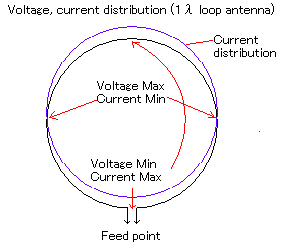# Principle and feature of loop antenna

(1/2)What makes the λ dipole aerial two step stack, connects the point mutually, and loops roundly is one wavelength (λ) loop antenna. It is thought that the line on falling the dead dipole is pulled and it made it to the circle. It is an antenna that it is easy and efficiency is good.

## What is the loop antenna?

The length of the element is with the antenna that the element of the antenna was the circle and there are various one like the long one and the short one, etc. with the loop antenna compared with wavelength.

It is called one wavelength (λ) loop antenna to be often used by the one that makes the (1/2) λ dipole aerial two step stack, connects the point mutually, and looped roundly.

Moreover, the one that the diameter rolled the lead several times very small compared with wavelength is called a loop antenna. Because this is the same as the coil, the magnetic field is used. It chiefly uses it for the reception.

Additionally, there is the one that the element duration that was called a minute loop antenna connected the capacitor below 1/4 wavelength on the opposite side of the feeding point, too. The minute loop antenna doesn't use the electric field, and it is an antenna using the magnetic field, and the directivity becomes right-angled direction compared with one wavelength loop. Efficiency doesn't at all reach one wavelength loop antenna though it is used also for the transmission of amateur radio.

## Feature of one wavelength (λ) loop antenna

Generally, the loop antenna used well is one wavelength (λ) loop antenna. When this principle is understood, various applications are possible.

### Length of one wavelength (λ) loop antenna(extension rate)

The loop antenna of 1λ is tuned though it shortens more than the wavelength calculated by using the wavelength shortening rate in the dipole aerial etc. and it uses it by an element duration that is a little longer than 1λ. It is necessary to lengthen the element at the extension rate of about 10 percent though it depends on the thickness of the element.

It is SWR(Standing Wave Ratio) to make it accurately To minimize this, the length of the element is adjusted measuring the standing-wave ratio with the meter. However, it is a good point hurried operating even if it suitably makes it.

### Voltage and current distribution of 1λ loop antennaThe size of the current of the current distribution is displayed by the distance from the element in pattern diagrams of a left one wavelength loop antenna.

It is a kind of the tune type antenna because it is thought that 1λ loop antenna stacked 1/2 wavelength dipole antenna. The voltage becomes on the other side of the feeding point and the feeding point and the currents become maximums lowest. And, the voltage is the maximum in the point where 1/4λ parts from the feeding point, and the current is minimized. The direction of the current becomes the same by the wise dispensation of Providence direction by both elements.

### Impedance of 1λ loop antenna

The feeding power point impedance of one wavelength loop antenna is called about 110Ω. Because the feeding power point impedance of folded-dipole is about 300Ω, the feeding power point impedance of one wavelength loop is easily as middle of 300Ω and 73Ω imaginable.

There was a twin loop antenna on two more stages stacked one wavelength loop antenna. This is what one wavelength loop antenna in parallel, the commonly used coaxial cable systems can be powered by 50Ω.

### Directivity and gain of 1λ loop antenna

If the above or below the feed point is the wave of horizontal polarization. The horizontal orientation at this time will be shaped 8.(like a 1/2λdipole)

Moreover, with the feeding point sideways, it becomes the electric wave of the vertically-polarized wave. The directivity in a horizontal plane at this time becomes a directivity that looks like two row stack of the vertical dipole.

Virtual antenna (Isotropc Antena) with respect to the gain will be approximately 4dBi. In comparison with the gain or the gain of the dipole will be approximately 2dBd.

Two element intervals narrow a little than the (1/2) λ, and the rise of the gain of 2dB is appropriate because the rise of the gain of generally two step-stack is 3dB in the calculation in case of one wavelength loop.

## Application of 1λ loop antenna

• Twin loop antenna has an antenna gain to feed the stack at the same time earn more than one loop antenna. It is used primarily for television transmission.
• Shaped antenna elements arranged in a loop around 1λ are easily made as a high gain yagi UHF is commonly used for sending and receiving. Add those two elements in particular reflector Qubical-Quad, saying the HF amateur radio antenna has been used since ancient times in the beam.
• The antenna that arranges the element of 1λ loop type back and forth, and fed power simultaneously includes the antenna named Swiss-Quad. This change is made to the loop antenna HB9CV antenna.
• There is a bird cage antenna in the one that Qubical-Quad was transformed, too.